Next: 4.2 The Quasi Full-Newton Up: 4.1 The Two-Level Newton Previous: 4.1.1 Calculation of Conductances

## 4.1.2 Modified Two-Level Newton Algorithm

A linear prediction step can be used to obtain an initial-guess from the previous solution. The two-level Newton scheme with a linear prediction step is referred to as the modified two-level Newton algorithm. A first-order prediction is made by use of the forward-Euler scheme,

 xk + 1 = xk +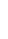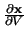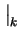.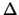V (4.12)

whereV is the change in voltage from circuit iteration k to k + 1, and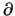x/V is calculated as in (4.8). In the investigations of  this algorithm had the most reliable convergence properties.

Tibor Grasser
1999-05-31